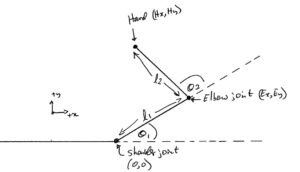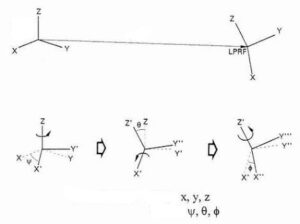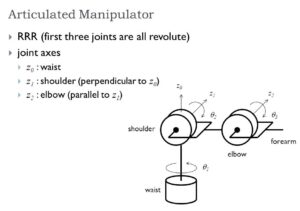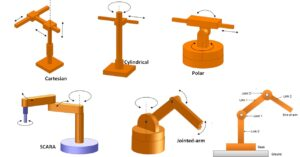# Robotics Technology

Robotics (Intro video)

IEEE RAS

A real scenario:  Underwater Robotics

Kinematics is the relationships between the positions, velocities, and accelerations of the links of a manipulator, where a manipulator is an arm, finger, or leg. In this chapter, we will develop a mathematical framework for describing these relationships, in particular position.

In the kinematic analysis of manipulator position, there are two separate problems to solve: direct kinematics, and inverse kinematics. Direct kinematics involves solving the forward transformation equation to find the location of the hand in terms of the angles and displacements between the links.

Introduction to Robotics John J. Craig 2013

www.roboanalyzer.com/

www.robodk.com/

Robot Kinematics Simulator

MatLab Simulink Toolbox Software for robotics and vision system

www.petercorke.com/Home.html  Prof.Peter Corke  (The University of Queensland – Australia)

3D model based software, Prof. S.K. Saha  (Dep.of Mechanical Eng, Indian Institute Of Technology Delhi IITDelhi)LecturesRPY angle sets

Euler angles

Rotation

ZYZ Rotation